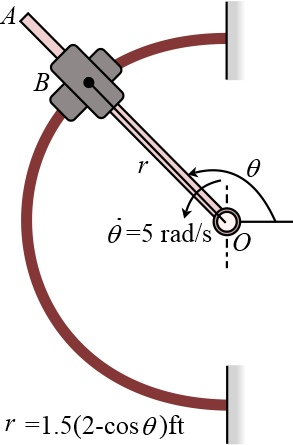# Rod OA rotates counterclockwise with a constant angular velocity of theta dot=5 rad/s. The double...

## Question:

Rod OA rotates counterclockwise with a constant angular velocity of {eq}\dot{\theta }=5\text{ rad/s} {/eq}. The double pin collar B is pin connected together such that one collar slides over the rotating rod and the other slides over the horizontal curved rod, of which the shape is described by the equation {eq}r=1.5\left( 2-\cos \theta \right)\text{ ft} {/eq}. If the both collars weigh 0.5 lbf, determine the normal force which the curve exerts on one collar at the instant {eq}\theta =120{}^\circ {/eq}. Negelct friction.## Reaction force

Reaction force is defined as the equal and opposite amount of force exerted by a body in response to an external force applied to the body. The standard unit of Reaction force is Newton.

Become a Study.com member to unlock this answer! Create your account

Given Data

• Angular velocity of the rod is {eq}\theta = 5\;{\rm{rad/s}} {/eq}

• Equation of the radius is {eq}= 1.5\left( {2 - \cos \theta }...Action and Reaction Forces: Law & Examples

from

Chapter 4 / Lesson 21
227K

Action force is force acting in one direction. Reaction force is force acting in the opposite direction. Learn more about Newton's Third Law as it explains action and reaction forces through several examples, and test your knowledge with quiz questions.